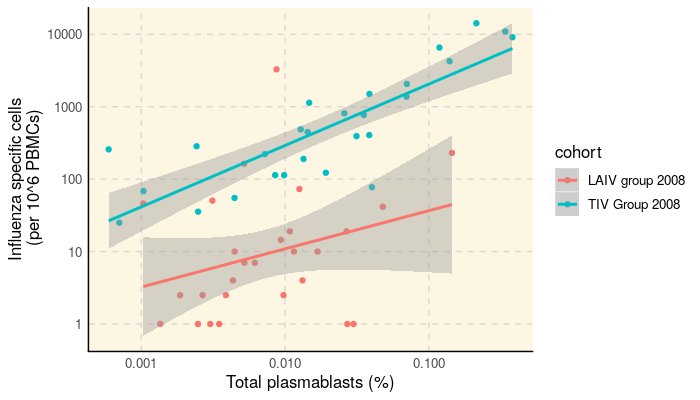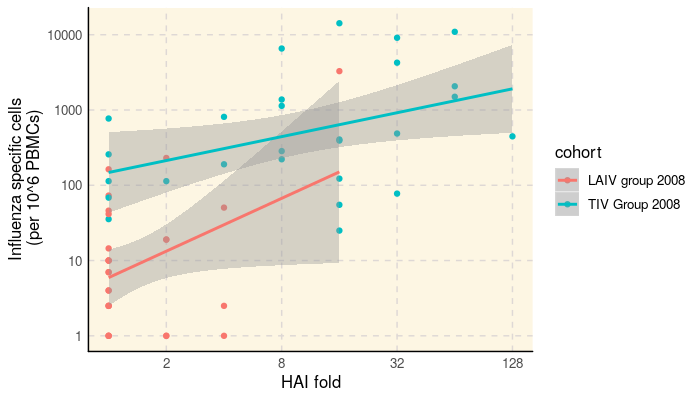SDY269: Correlating HAI with Flow Cytometry and ELISPOT Results

2019-07-09

ImmuneSpaceR code produces consistent results, regardless of whether it is being executed from a module or UI based report on the server or on a local machine. This vignette reproduces a report available on the ImmuneSpace portal using the same code.

1 Summary

This report investigate the association between the number influenza-specific cells measured by ELISPOT measured at day 7 with the number of plasmablast measured by flow cytometry and day 7 and the HAI response measured at day 28 (log-fold day28/day0). It basically reproduces Figure 1 d-e) of Nakaya et al. (2011) published as part of the original study.

2 Load ImmuneSpaceR and other libraries

library(ImmuneSpaceR)
library(ggplot2)
library(data.table)

5 Merge data and phenodata

# Let's key the different datasets
setkeyv(dt_hai, c("participant_id"))
setkeyv(dt_fcs, c("participant_id"))
setkeyv(dt_elispot, c("participant_id"))
dt_all <- dt_hai[dt_fcs, nomatch = 0][dt_elispot, nomatch = 0]

The figure below shows the absolute number of plasmablast cells measured by flow cytometry vs. the number of frequency of influenza-specific cells measured by ELISPOT.

ggplot(dt_all, aes(x = as.double(fcs_response), y = elispot_response, color = cohort)) +
geom_point() +
scale_y_log10() +
scale_x_log10() +
geom_smooth(method = "lm") +
xlab("Total plasmablasts (%)") +
ylab("Influenza specific cells\n (per 10^6 PBMCs)") +
theme_IS()The figure below shows the HAI fold increase over baseline vs. the number of frequency of influenza-specific cells measured by ELISPOT.

ggplot(dt_all, aes(x = as.double(hai_response), y = elispot_response, color = cohort)) +
geom_point() +
scale_x_continuous(trans = "log2") +
scale_y_log10() +
geom_smooth(method = "lm") +
xlab("HAI fold") +
ylab("Influenza specific cells\n (per 10^6 PBMCs)") +
theme_IS()In each case, we observe good correlations between the different responses, at least for the TIV cohort.